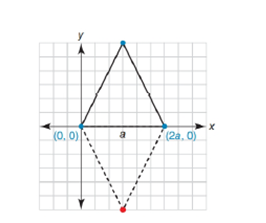Chapter 10.1, Problem 29EElementary Geometry For College St...

7th Edition
Alexander + 2 others
ISBN: 9781337614085

Solutions

Chapter
SectionElementary Geometry For College St...

7th Edition
Alexander + 2 others
ISBN: 9781337614085
Textbook Problem

If two vertices of an equilateral triangle are at ( 0, 0 ) and ( 2 a ,   0 ) , what is the third vertex?To determine

What is the third vertex, if two vertices of an equilateral triangle are at (0, 0) and (2a, 0).

Explanation

Given vertices are,

(0, 0) and (2a, 0)

Given figure is,

Let the given triangle is ABC.

Length of AB =a

Coordinates of A=(0, 0)

Coordinates of B=(2a, 0)

Let the coordinates of C=(x, y)

Since, the given triangle is an equilateral, the length of three sides are equal.

AB=BC=CA=a

a can be determined using distance formula as below,

Distance Formula:

The distance between the two points (x1, y1) and (x2, y2) as below,

d=(x2-x1)2+(y2-y1)2

Coordinates of A(0, 0), B(2a, 0)

Length of MN=(2a-0)2+(0-0)2

Length of MN=(2a)2

Length of MN=2a

Coordinates of C can be determined from the length of AC and BC

A=(0, 0) and C=(x, y)

Length of AC=(x-0)2+(y-0)2

Length of AC=(x)2+(y)2=2a

Still sussing out bartleby?

Check out a sample textbook solution.

See a sample solution

The Solution to Your Study Problems

Bartleby provides explanations to thousands of textbook problems written by our experts, many with advanced degrees!

Get Started

Prove Equation 4.

Single Variable Calculus: Early Transcendentals, Volume I

In Exercises 2124, factor each expression completely. 21. 22r3 + 100r2

Applied Calculus for the Managerial, Life, and Social Sciences: A Brief Approach

Find the value of k such that (k,k) is equidistant from (1,0) and (0,2).

Finite Mathematics and Applied Calculus (MindTap Course List)

The critical numbers of f(x) = 3x4 = 20x3 36x2 are: a) 0, 1, 6 b) 0, 1, 6 c) 0, 1, 6 d) 0, 1, 6

Study Guide for Stewart's Single Variable Calculus: Early Transcendentals, 8th

Define applied research and basic research and identify examples of each.

Research Methods for the Behavioral Sciences (MindTap Course List)